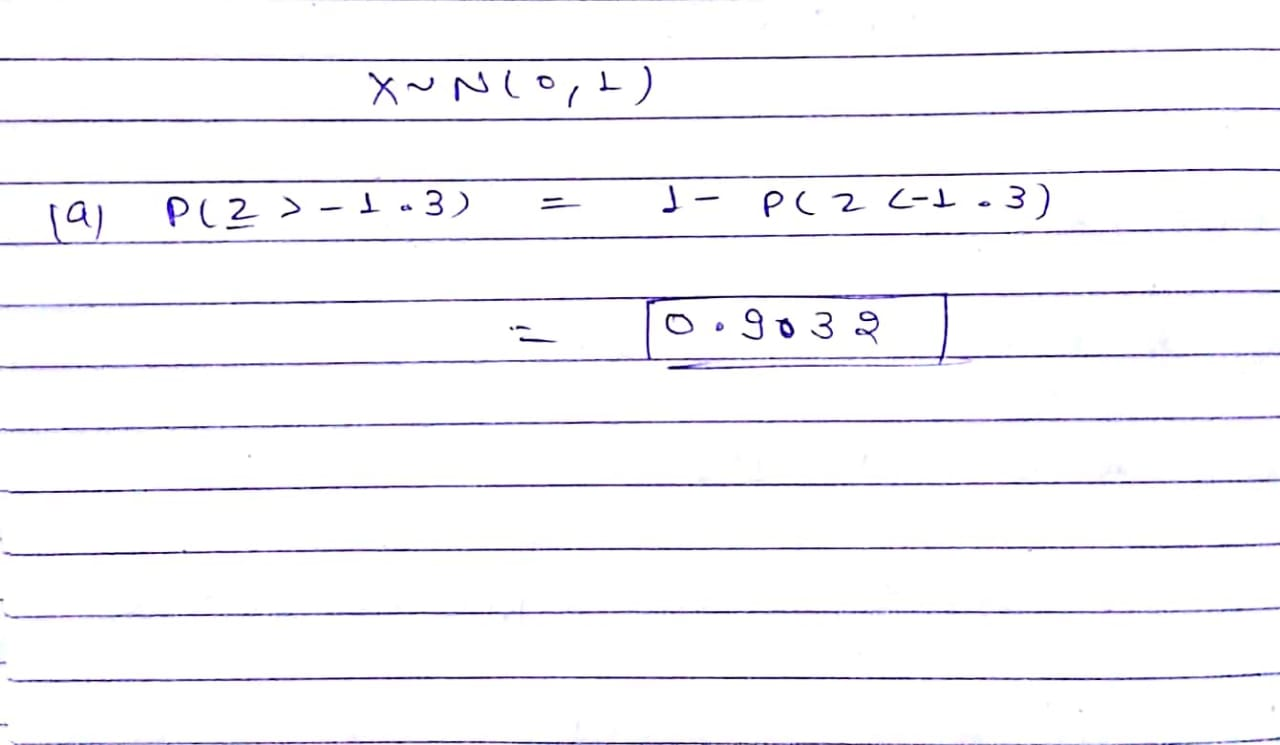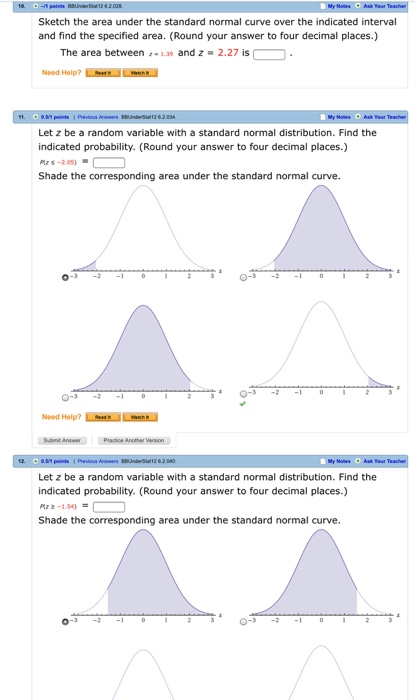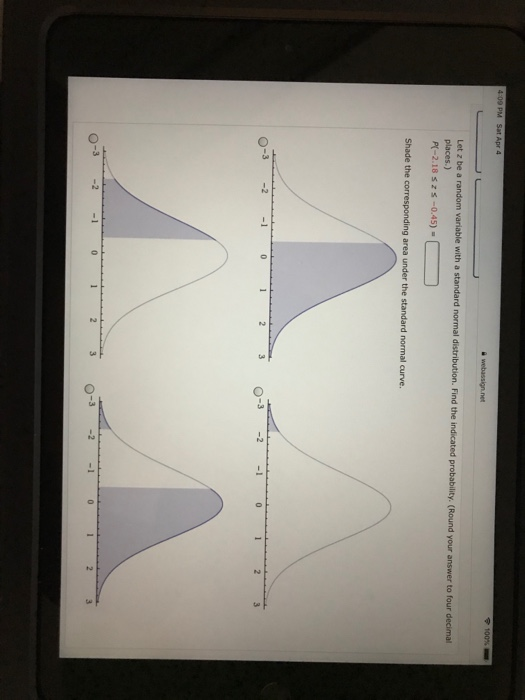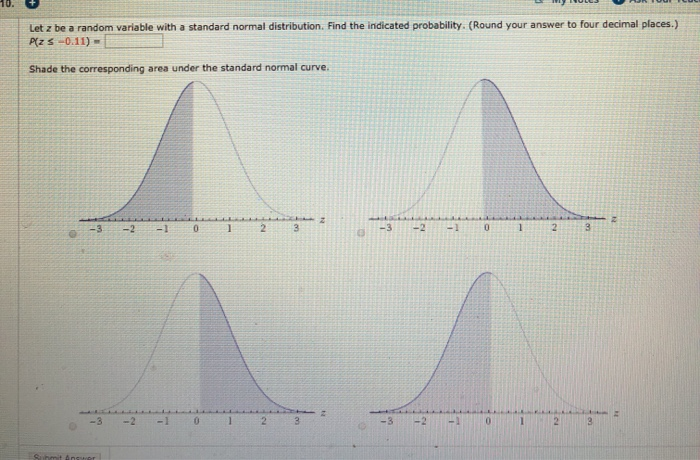Question

# Please show step by step and how to enter in the calculator Find the indicated probability...

Please show step by step and how to enter in the calculator

Find the indicated probability given that Z is a random variable with a standard normal distribution. (Round your answer to four decimal places.)

P(Z ≥ -1.3) P(Z ≥ -1.3) =#### Earn Coins

Coins can be redeemed for fabulous gifts.

Similar Homework Help Questions
• ### Let z be a random variable with a standard normal distribution. Find the indicated probability. (Enter...

Let z be a random variable with a standard normal distribution. Find the indicated probability. (Enter a number. Round your answer to four decimal places.) P(z ≥ 1.41) =

• ### Find the indicated probability given that Z is a random variable with a standard normal distribution....

Find the indicated probability given that Z is a random variable with a standard normal distribution. (Round your answer to four decimal places.) P(0 ≤ Z ≤ 1.28) P(0 ≤ Z ≤ 1.28) =

• ### Sketch the area under the standard normal curve over theindicated interval and find the specified...Sketch the area under the standard normal curve over the indicated interval and find the specified area. (Round your answer to four decimal places.)The area betweenz = 1.39and z = 2.27 is .Let z be a random variable with a standard normal distribution. Find the indicated probability. (Round your answer to four decimal places.)P(z ≤ −2.05)=   Let z be a random variable with a standard normal distribution. Find the indicated probability. (Round your answer to four decimal places.)P(z ≥ −1.54)=...

• ### Let z be a random variable with a standard normal distribution. Find the indicated probability. (Round...

Let z be a random variable with a standard normal distribution. Find the indicated probability. (Round your answer to four decimal places.) P(z ≤ −1.94) = [x].

• ### Let z be a random variable with a standard normal distribution. Find the indicated probability. (Round...

Let z be a random variable with a standard normal distribution. Find the indicated probability. (Round your answer to four decimal places.) P(−1.24 ≤ z ≤ 2.64) = Shade the corresponding area under the standard normal curve.

• ### Let z be a random variable with a standard normal distribution. Find the indicated probability. (Round...

Let z be a random variable with a standard normal distribution. Find the indicated probability. (Round your answer to four decimal places.) P(z ≤ −0.11) = P(z ≥ 1.25) = P(−1.17 ≤ z ≤ 2.44) = P(0 ≤ z ≤ 1.65) =

• ### 100% Let z be a random variable with a standard normal distribution. Find the indicated probability....100% Let z be a random variable with a standard normal distribution. Find the indicated probability. (Round your answer to four decimal places.) P(-2.18 SZS -0.45) - Shade the corresponding area under the standard normal curve.

• ### Let z be a random variable with a standard normal distribution. Find the indicated probability. (Round...

Let z be a random variable with a standard normal distribution. Find the indicated probability. (Round your answer to four decimal places.) P(−2.02 ≤ z ≤ −0.40) = Shade the corresponding area under the standard normal curve. WebAssign Plot WebAssign Plot WebAssign Plot WebAssign Plot

• ### Let z be a random variable with a standard normal distribution. Find the indicated probability. (Round...Let z be a random variable with a standard normal distribution. Find the indicated probability. (Round your answer to four decimal places.) PzS -0.11) Shade the corresponding area under the standard normal curve - 1 2 3 -3 -2 -

• ### Let z be a random variable with a standard normal distribution. Find the indicated probability. (Round...

Let z be a random variable with a standard normal distribution. Find the indicated probability. (Round your answer to four decimal places.) P(z ≤ −0.22)= P(z ≤ 1.18)= P(z ≤ 3.02)= P(z ≥ 1.90)= P(z ≥ −1.59)= P(−1.24 ≤ z ≤ 2.71)= P(−2.13 ≤ z ≤ 1.01)= P(−2.04 ≤ z ≤ −0.40)= P(−1.90 ≤ z ≤ −1.17)= P(0 ≤ z ≤ 1.52)= P(−0.84 ≤ z ≤ 0)=